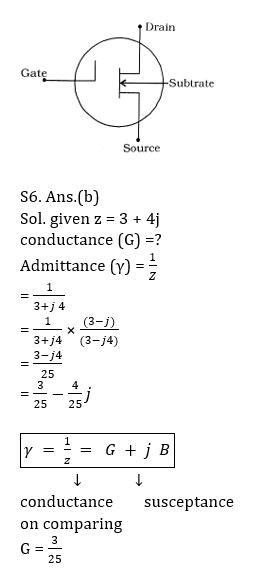Engineering Jobs   »   uprvunl je electrical quiz,

# UPRVUNL’21 EE: Daily Practices Quiz 16-Aug-2021

Each question carries 1 mark.
Negative marking: 1/4 mark
Total Questions: 6
Time: 6 min.

Q1. Pole mounted substations are used in
(a) Primary distribution system
(b) Secondary distribution system
(c) Primary transmission system
(d) Secondary transmission system

Q2. Under running conditions, the maximum torque of 3-phase induction motor will
occur at that value of slip at which rotor resistance/phase is ……. rotor reactance/phase.
(a) two times
(b) four times
(c) equal to
(d) none of the above

Q3. An 8-pole alternator runs at 750 r.p.m. and supplies power to a 6-pole induction
motor which has a full-load slip of 3%. The full-load speed of the motor is
(a) 1050 r.p.m.
(b) 970 r.p.m.
(c) 960 r.p.m.
(d) 1250 r.p.m.

Q4. The two-part tariff is used for…………consumers.
(a) Agricultural
(b) Commercial
(c) Domestic
(d) Industrial

Q5. A MOSFET has………terminals.
(a) two
(b) five
(c) four
(d) three

Q6. The impedance of a circuit is given by Z = 3 + j4. its conductance will be:
(a) 3/4
(b) 3/25
(c) 1/3
(d) 3/7

SOLUTIONS

S1. Ans.(b)
Sol. Pole mounted substations:
Used in secondary distribution system.
It is an outdoor substation with equipment installed overhead on H-type or 4-pole structure.
The voltage rating of transformers in a pole mounted substations is 11 KV/400 V.

S2. Ans. (c)
Sol. The developed torque is maximum when the rotor resistance per phase is equal to the rotor reactance per phase under running conditions.

S3. Ans.(b)
Sol. An 8-pole alternator runs at 750 r.p.m. and supplies power to induction
Motor.
⇒f=50 Hz
Speed of stator flux of induction motor
=120f/P=(120×50)/6
= 1000 rpm
∴N_r=(1-s) N_s
=(1-0.03)×1000
= 970rpm

S4. Ans.(d)
Sol. The two-part tariff is used for industrial consumers. In the two-part tariff, there are fixed charge and running charge which depends on maximum demand and total consumed electrical energy respectively.

S5. Ans.(d)
Sol. Schematic symbol of n-channel MOSFET:Sharing is caring!